CBSE Class 10 Sample Paper for 2021 Boards - Maths Standard

Class 10
Solutions of Sample Papers for Class 10 Boards

## Draw a line segment AB of length 9cm. With A and B as centres, draw circles of radius 5cm and 3cm respectively. Construct tangents to each circle from the centre of the other circle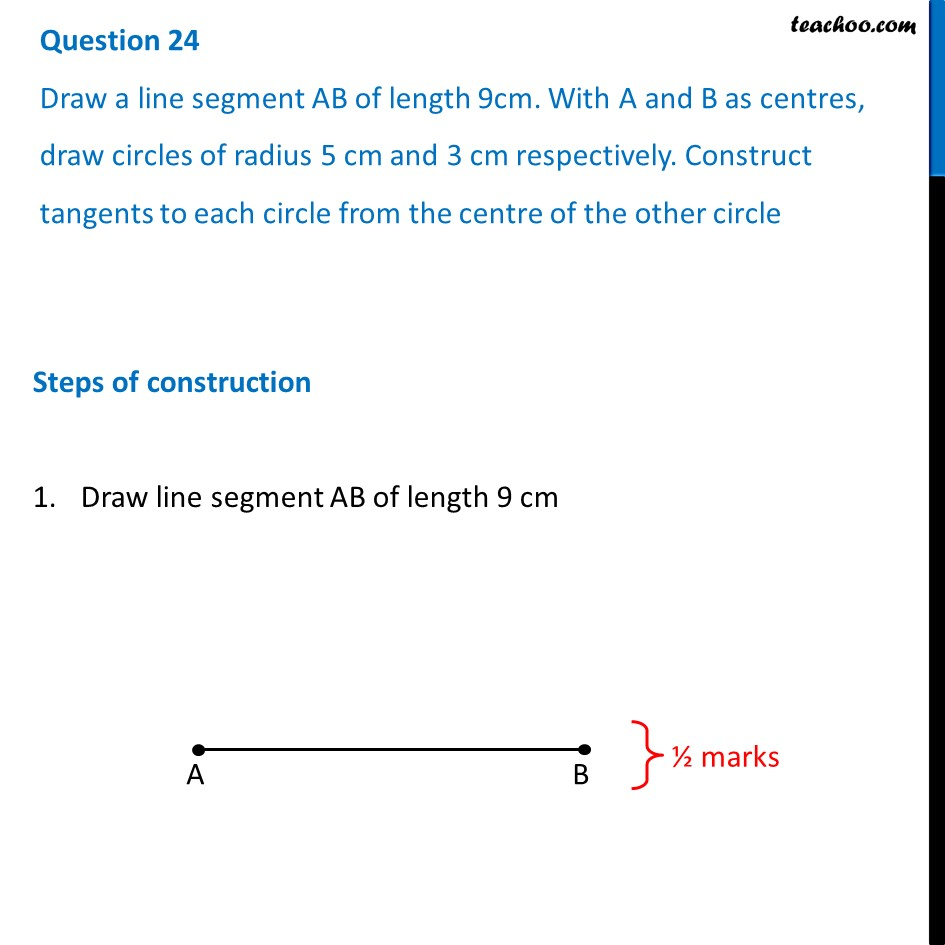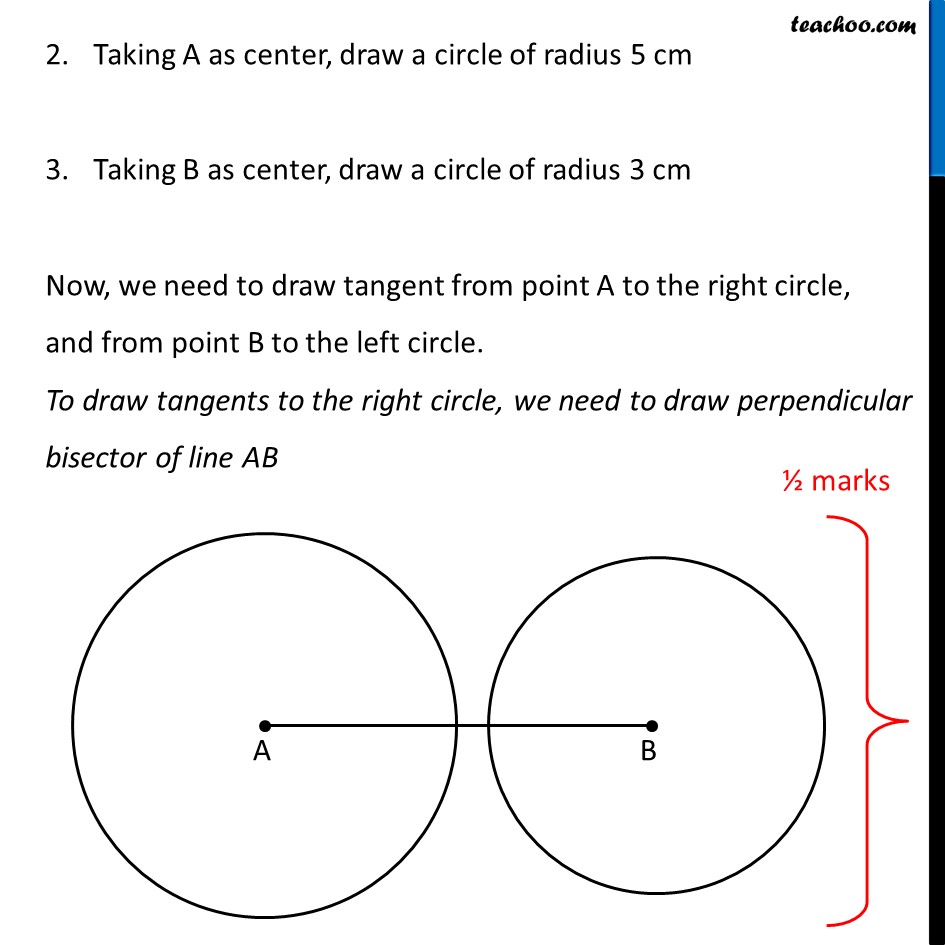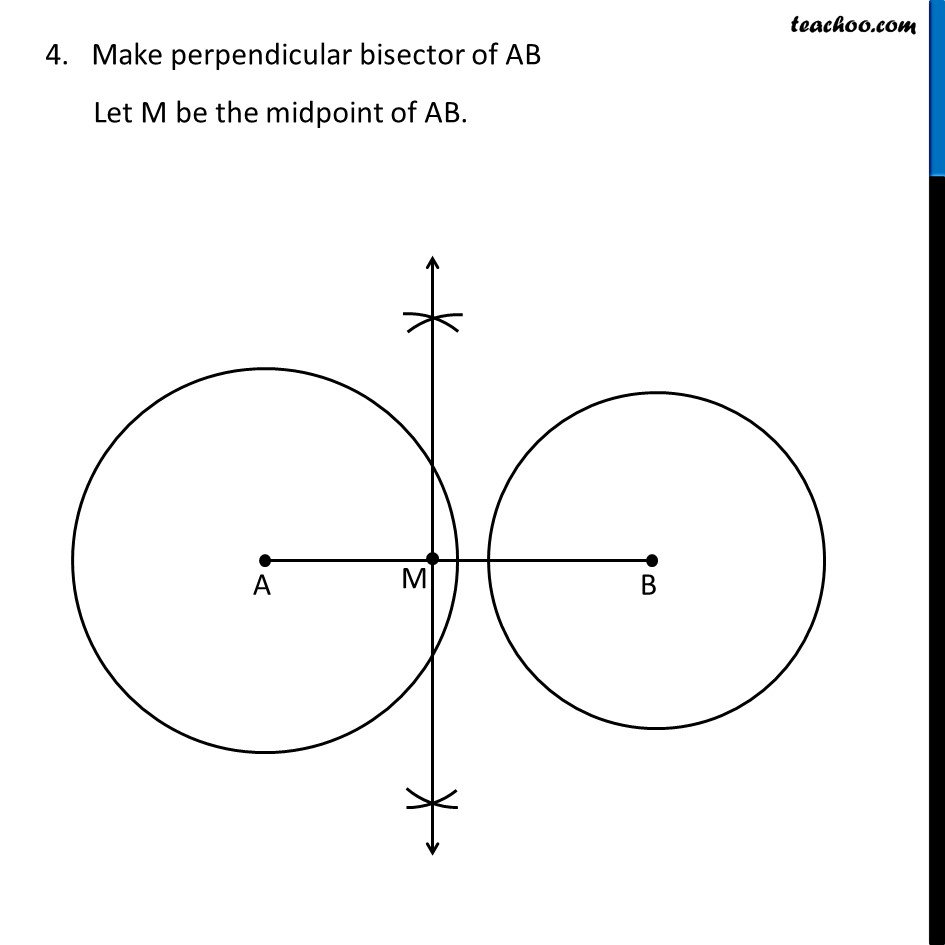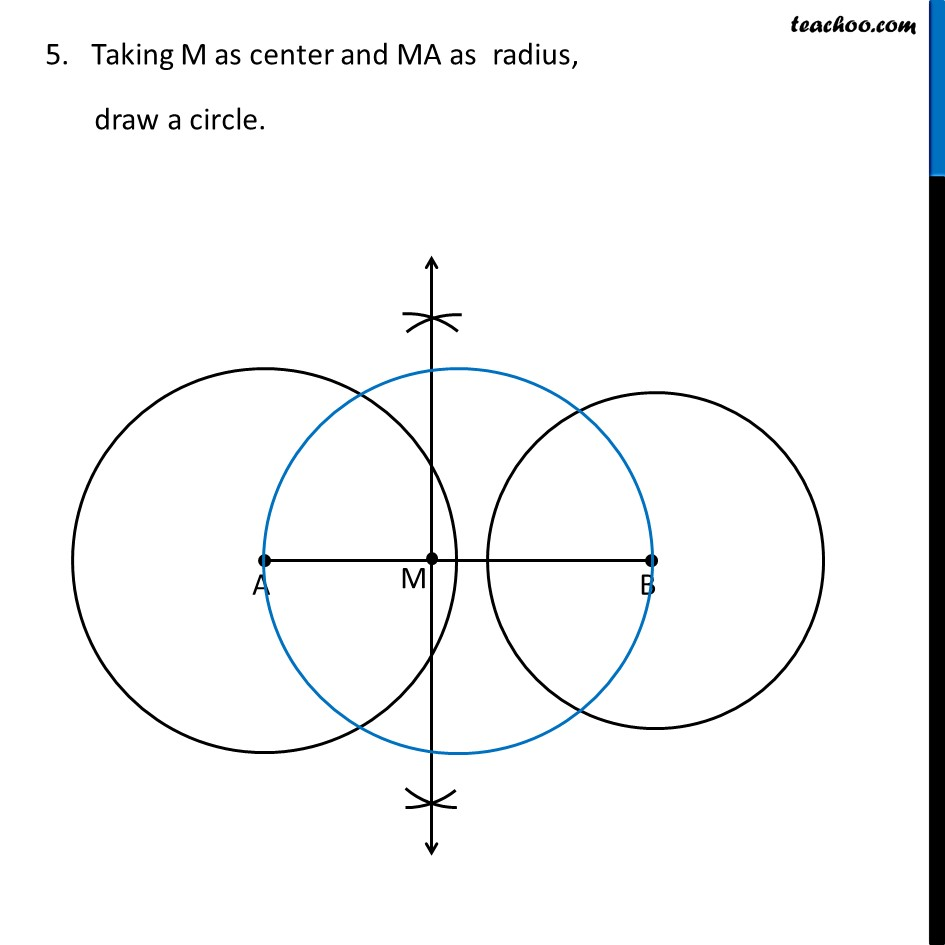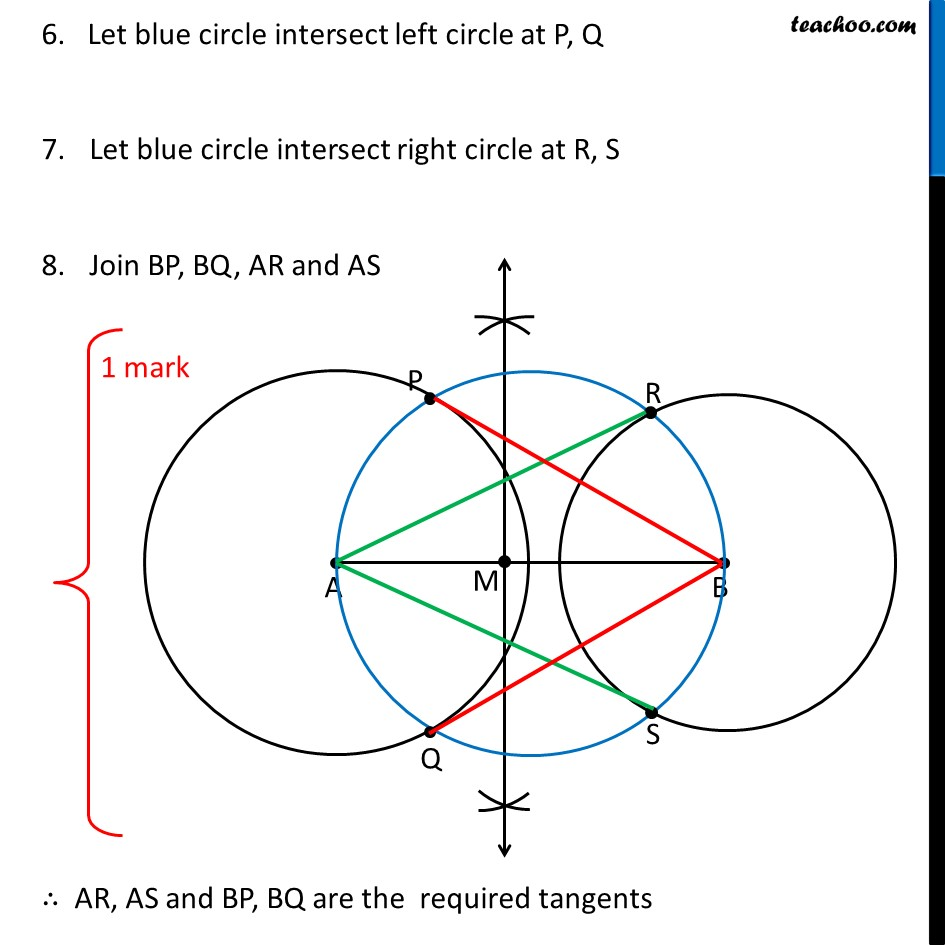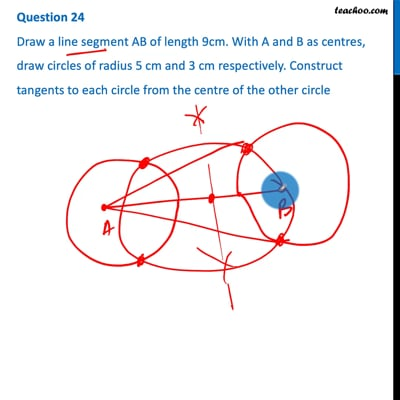This video is only available for Teachoo black users

Note : This is similar to Ex 11.2, 5 of NCERT – Chapter 11 Class 10 Constructions

Check the answer here https:// www.teachoo.com /5755/543/Ex-11.2--5---Draw-a-line-segment-AB-of-length-8-cm.-Taking-A-as-centre/category/Ex-11.2/

Introducing your new favourite teacher - Teachoo Black, at only ₹83 per month

### Transcript

Question 24 Draw a line segment AB of length 9cm. With A and B as centres, draw circles of radius 5 cm and 3 cm respectively. Construct tangents to each circle from the centre of the other circle Steps of construction Draw line segment AB of length 9 cm Taking A as center, draw a circle of radius 5 cm Taking B as center, draw a circle of radius 3 cm Now, we need to draw tangent from point A to the right circle, and from point B to the left circle. To draw tangents to the right circle, we need to draw perpendicular bisector of line AB Make perpendicular bisector of AB Let M be the midpoint of AB. Taking M as center and MA as radius, draw a circle. 6. Let blue circle intersect left circle at P, Q Let blue circle intersect right circle at R, S Join BP, BQ, AR and AS ∴ AR, AS and BP, BQ are the required tangents